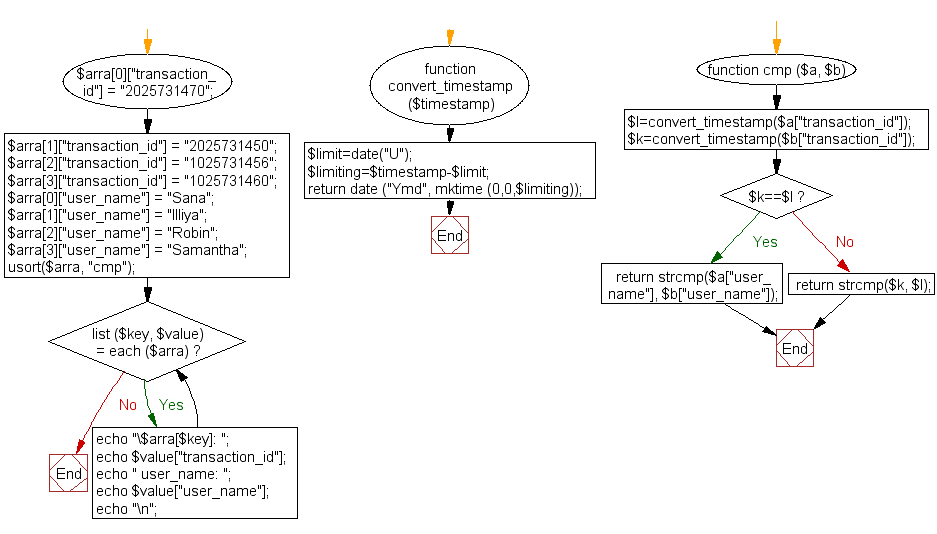﻿ PHP Array Exercise: Sort the following array by the day and username - w3resource# PHP Array Exercises : Sort a specified array by the day and username

## PHP Array: Exercise-22 with Solution

Write a PHP script to sort the following array by the day (page_id) and username.

Sample Solution:

PHP Code:

``````<?php
\$arra["transaction_id"] = "2025731470";
\$arra["transaction_id"] = "2025731450";
\$arra["transaction_id"] = "1025731456";
\$arra["transaction_id"] = "1025731460";
\$arra["user_name"] = "Sana";
\$arra["user_name"] = "Illiya";
\$arra["user_name"] = "Robin";
\$arra["user_name"] = "Samantha";

//convert timestamp to date
function convert_timestamp(\$timestamp){
\$limit=date("U");
\$limiting=\$timestamp-\$limit;
return date ("Ymd", mktime (0,0,\$limiting));
}
//comparison function
function cmp (\$a, \$b) {
\$l=convert_timestamp(\$a["transaction_id"]);
\$k=convert_timestamp(\$b["transaction_id"]);
if(\$k==\$l){
return strcmp(\$a["user_name"], \$b["user_name"]);
}else{
return strcmp(\$k, \$l);
}
}
//sort array
usort(\$arra, "cmp");

//print sorted info
while (list (\$key, \$value) = each (\$arra)) {
echo "\\$arra[\$key]: ";
echo \$value["transaction_id"];
echo " user_name: ";
echo \$value["user_name"];
echo "\n";
}
?>
```
```

Sample Output:

```\$arra: 2025731450 user_name: Illiya
\$arra: 2025731470 user_name: Sana
\$arra: 1025731456 user_name: Robin
\$arra: 1025731460 user_name: Samantha
```

Flowchart:PHP Code Editor:

What is the difficulty level of this exercise?

Test your Programming skills with w3resource's quiz.

﻿

## PHP: Tips of the Day

Concatenation Operators: You can use concatenation to join strings "end to end" while outputting them (with echo or print).

You can concatenate variables using a . (period/dot).

Example:

```<?php
// String variable

\$name = 'Jhon';

// Concatenate multiple strings (3 in this example) into one and echo it once done.

echo '<p>Hello ' . \$name . ', Nice to meet you.</p>';

// Concatenation Operators
?>
```

Output:

```<p>Hello Jhon, Nice to meet you.</p>
```

Similar to concatenation, echo (when used without parentheses) can be used to combine strings and variables together (along with other arbitrary expressions) using a comma (,).

```<?php
\$itemCount = 1;
echo 'You have learn ', \$itemCount, ' Tips', \$itemCount === 1 ? '' : 's';
?>
```

Output:

```You have learn 1 Tips
```

String concatenation vs passing multiple arguments to echo

Passing multiple arguments to the echo command is more advantageous than string concatenation in some circumstances. The arguments are written to the output in the same order as they are passed in.

```echo "The total is: ", \$x + \$y;
```

The problem with the concatenation is that the period . takes precedence in the expression. If concatenated, the above expression needs extra parentheses for the correct behavior. The precedence of the period affects ternary operators too.

```echo "The total is: " . (\$x + \$y);
```